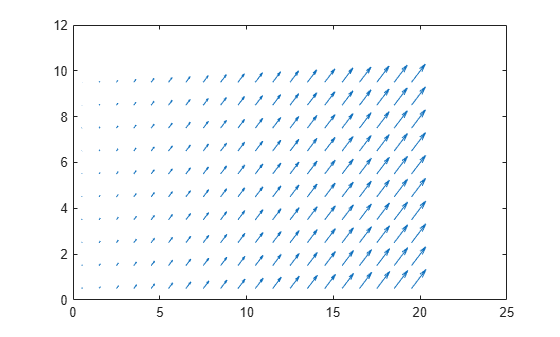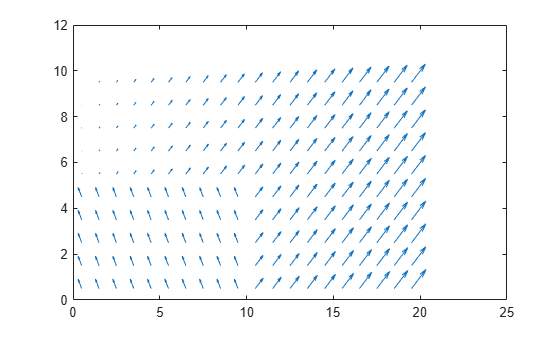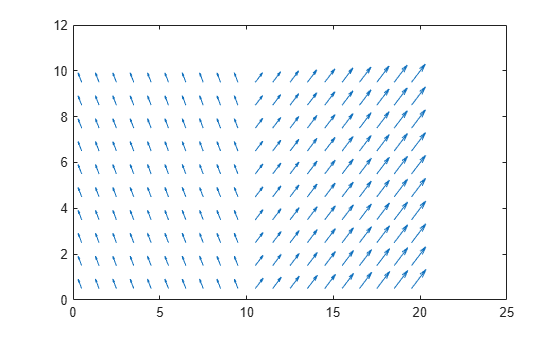# mapLayer

Create map layer for N-dimensional data

## Description

The `mapLayer` object creates an N-dimensional grid map, where the first two dimensions determine the footprint of the map, and all subsequent dimensions dictate the size and layout of the data stored in each cell. For storing scalar binary or probability values for a grid map, use the `binaryOccupancyMap` or `occupancyMap` objects instead.

A map layer stores data for grid cells that represent a discretized region of space. To query and update data using world, local, or grid coordinates, use the `getMapData` and `setMapData` object functions. Each grid cell in the map can store data of any size from a single a value to a multi-dimensional array. For more information, see the DataSize property.

Layer behavior can also be customized by providing function handles during creation using the `GetTransformFcn` and `SetTransformFcn` properties.

## Creation

### Syntax

``map = mapLayer``
``map = mapLayer(p)``
``map = mapLayer(width,height)``
``map = mapLayer(rows,cols,'grid')``
``map = mapLayer(width,height,cellDims)``
``map = mapLayer(rows,cols,cellDims,'grid')``
``map = mapLayer(sourceMap)``
``map = mapLayer(___,Name,Value)``

### Description

````map = mapLayer` creates an empty map object occupying 10-by-10 meters of space with a resolution of 1 cell per meter.```
````map = mapLayer(p)` creates a map from the values in the matrix or matrix array `p`. For 3-D matrix arrays, each cell in the map is filled with the vector of values at each grid location along the third dimension of the array. For an N-by-D matrix array, each cell contains a matrix (N=4) or a matrix array (N>4) of data for that grid location.```
````map = mapLayer(width,height)` creates a map covering the specified width and height with a resolution of 1 cell per meter.```
````map = mapLayer(rows,cols,'grid')` creates a map with a grid size of `rows`,`cols` with a resolution of 1 cell per meter.```
````map = mapLayer(width,height,cellDims)` creates the map where the size of the data stored in each cell is defined by the array of integers `cellDims`.```
````map = mapLayer(rows,cols,cellDims,'grid')` creates a map with a grid size of `rows``cols` where the size of the data stored in each cell is defined by the array of integers `cellDims`.```
````map = mapLayer(sourceMap)` creates a new object using the layers copied from another `mapLayer` object.```

example

````map = mapLayer(___,Name,Value)` specifies property values using name-value pairs. For example, `mapLayer(__,'LocalOriginInWorld',[15 20])` sets the local origin to a specific world location.```

## Properties

expand all

Size of the N-dimensional data matrix, specified as vector of integers. The first two dimensions define the footprint of the map, and all subsequent dimensions dictate the size and layout of the data stored in each cell. The default value assumes a single value is stored for each cell in a 10-by-10 grid.

If the map stores an n-element vector of values in each cell, this property would be `[width height n]`.

If the map stores a 10-by-10 grid with each cell containing a 3-by-3-by-3 matrix array, the data size would be `[10 10 3 3 3]`.

This property is set when you create the object based on the dimensions of the input matrix `p` or the inputs `width`, `height`, and `cellDims`.

After you create the object, this property is read-only.

Data Types: `double`

Data type of the values stored in the map, specified as a character vector.

This property is set based on the data type of the input `p` or the data type of DefaultValue. After you create the object, this property is read-only.

Data Types: `char`

Default value for unspecified map locations including areas outside the map, specified as a numeric scalar.

If you specify the GetTransformFcn or SetTransformFcn property when creating the object, the default value is updated based on that transformation function. If you create the map with a matrix of values `p`, the transform function modifies the values before storing.

Data Types: `double`

Applies transformations to values retrieved by the `getMapData` function, specified as a function handle.

This function handle is called inside the `getMapData` object function. It can be used to apply a transformation to values retrieved from the map layer. The function definition must have the following format:

`modifiedValues = getTransformFcnHandle(map,values,varargin)`

The size of the output `modifiedValues` must match the size of the input `values`. The function provides all map data accessed from the `getMapData` object function to this transform function through the `varargin` inputs.

You can set this property when you create the object. After you create the object, this property is read-only.

Data Types: `function_handle`

Location of the bottom-left corner of the grid in world coordinates, specified as a two-element vector, `[xWorld yWorld]`.

You can set this property when you create the object.

Data Types: `double`

Location of the bottom-left corner of the grid in local coordinates, specified as a two-element vector, `[xLocal yLocal]`.

You can set this property when you create the object.

Data Types: `double`

Number of rows and columns in grid, stored as a 1-by-2 real-valued vector representing the number of rows and columns, in that order.

This property is set when you create the object based on the first two dimensions of the input matrix `p`, the inputs `width` and `height`, or the inputs `row` and `col`.

Data Types: `double`

Name of map layer, specified as a character vector or string scalar.

You can set this property when you create the object. After you create the object, this property is read-only.

Data Types: `double`

Location of the origin of the local frame in world coordinates, specified as a two-element vector, `[xLocal yLocal]`. Use the `move` function to shift the local frame as your vehicle moves.

You can set this property when you create the object.

Data Types: `double`

Grid resolution, stored as a scalar in cells per meter representing the number and size of grid locations.

You can set this property when you create the object. After you create the object, this property is read-only.

Data Types: `double`

Applies transformations to values set by the `setMapData` function, specified as a function handle.

This function handle is called inside the `setMapData` object function. It can be used to apply a transformation to values set in the map layer. The function must have the following syntax:

```modifiedValues = setTransformFcnHandle(map,values,varargin) if numel(varargin) == 0 return; % else % Custom Code end end ```

The size of the output, `modifiedValues`, must match the size of the input, `values`. The function provides all map data specified in the `setMapData` object function to this transform function. When creating this object without starting values, the function is called without additional input arguments, so specify an if-statement to return when the number of elements in `varagin` is zero.

You can set this property when you create the object. After you create the object, this property is read-only.

Data Types: `function_handle`

Minimum and maximum values of x-coordinates in local frame, stored as a two-element horizontal vector of the form `[min max]`. Local frame is defined by `LocalOriginInWorld` property.

Data Types: `double`

Minimum and maximum values of y-coordinates in local frame, stored as a two-element horizontal vector of the form `[min max]`. Local frame is defined by `LocalOriginInWorld` property.

Data Types: `double`

Minimum and maximum world range values of x-coordinates, stored as a 1-by-2 vector representing the minimum and maximum values, in that order.

Data Types: `double`

Minimum and maximum world range values of y-coordinates, stored as a 1-by-2 vector representing the minimum and maximum values, in that order.

Data Types: `double`

## Object Functions

 `getMapData` Retrieve data from map layer `grid2local` Convert grid indices to local coordinates `grid2world` Convert grid indices to world coordinates `local2grid` Convert local coordinates to grid indices `local2world` Convert local coordinates to world coordinates `move` Move map in world frame `setMapData` Assign data to map layer `syncWith` Sync map with overlapping map `world2grid` Convert world coordinates to grid indices `world2local` Convert world coordinates to local coordinates

## Examples

collapse all

Create a map layer that stores two values per grid location as xy-velocities.

Create an m-by-n-by-2 matrix of values. The first element in the third dimension is dx and the second is dy as velocities.

```dXY = reshape(1:200,10,20); dXY(:,:,2) = dXY;```

Create a map layer from the matrix. Specify the resolution and layer name.

`vLayer = mapLayer(dXY,'Resolution',1,'LayerName','dXY');`

Get all the map data out as a matrix. Get the xy-locations of the velocity values by creating arrays that cover the minimum and maximum xy-world limits and is shifted to the grid-center locations. The y-locations are flipped when converting between matrix to world coordinates. Visualize the velocities corresponding to those grid-center locations using the `quiver` function.

```v = getMapData(vLayer); R = 1/(2*vLayer.Resolution); xLim = vLayer.XWorldLimits; yLim = vLayer.YWorldLimits; xLoc = (xLim(1)+R):R*2:(xLim(2)-R); yLoc = (yLim(2)-R):-R*2:(yLim(1)+R); quiver(xLoc,yLoc,v(:,:,1),v(:,:,2))```Set the bottom-left quadrant to new updated values. Create the values as a matrix and specify the bottom-left corner (0,0) in map coordinates to the `setData` function.

```updateValues = repmat(reshape([-50,100],[1 1 2]),5,10); setMapData(vLayer,[0 0],updateValues) v = getMapData(vLayer); quiver(xLoc,yLoc,v(:,:,1),v(:,:,2))```Set new values for the top-left quadrant using grid coordinates. For maps, the top-left grid location is (1,1).

```setMapData(vLayer,[1 1],updateValues,'grid') v = getMapData(vLayer); quiver(xLoc,yLoc,v(:,:,1),v(:,:,2))```The `mapLayer` object enables you to apply custom element-wise transformations when setting and getting data in the map. To transform data you set or get from the map, specify function handles for the `GetTransfomFcn` and `SetTransformFcn `properties. This example shows how to implement a log-odds probabilitistic map layer by creating a lookup table for probability and log-odds values. The transform functions use these lookup tables to convert between these values when setting or getting data.

Create Lookup Tables

Generate a full lookup table of values that map the probability values to the minimum and maximum limits of `int16` values.

Create an array of `int16` values from `intmin` to `intmax`. Define the probablilty limits.

```intType = 'int16'; intLinSpace = intmin(intType):intmax(intType); numOfPoints = length(intLinSpace); probLimits = [0.001 .999];```

The `exampleHelperProbToLogodds` and `examplerHelperLogoddsToProb` functions covert between the log-odds and probability values. Use the helper functions to get the log-odds limits and generate the array for looking up log-odds values. Create an interpolated grid for the entire lookup table.

```logOddsLimits = exampleHelperProbToLogodds([0.001 .999]); logOddsLookup = single(exampleHelperLogoddsToProb(linspace(logOddsLimits(1),logOddsLimits(2),numOfPoints))); interpTable = griddedInterpolant(logOddsLookup,single(intLinSpace),'nearest');```

Specify Transform Function Handles

The transform function handles utilize example helpers that define how to convert between log-odds integer values and the probability values with an applied saturation limit. The probability saturation limits are `[0.001 .999]` as previously specified. This behavior is similar to the `occupancyMap` object.

```getXformFcn = @(obj,logodds,varargin)... exampleHelperIntLogoddsToProb(logodds,logOddsLookup(:),intLinSpace); setXformFcn = @(obj,prob,varargin)... exampleHelperProbToIntLogodds(prob,interpTable,logOddsLookup(:),intLinSpace,probLimits);```

Create Map Layer

Generate an occupancy map layer object from a matrix of probability values. Specify the get and set transform functions.

```occupancyLayer = mapLayer(repmat(0.5,10,10),... 'LayerName','Occupancy',... 'GetTransformFcn',getXformFcn,... 'SetTransformFcn',setXformFcn);```

Notice that when you create the map, the default value is 0.001 instead of 0. This difference is because the `SetTransformFcn` function has been applied to the default value of 0 when you create the object, which saturates the value to 0.001.

`disp(occupancyLayer.DefaultValue)`
``` 0.0010 ```

Get and Set Map Data

The map data matches the matrix you set on creation.

`extData = getMapData(occupancyLayer) `
```extData = 10×10 0.5000 0.5000 0.5000 0.5000 0.5000 0.5000 0.5000 0.5000 0.5000 0.5000 0.5000 0.5000 0.5000 0.5000 0.5000 0.5000 0.5000 0.5000 0.5000 0.5000 0.5000 0.5000 0.5000 0.5000 0.5000 0.5000 0.5000 0.5000 0.5000 0.5000 0.5000 0.5000 0.5000 0.5000 0.5000 0.5000 0.5000 0.5000 0.5000 0.5000 0.5000 0.5000 0.5000 0.5000 0.5000 0.5000 0.5000 0.5000 0.5000 0.5000 0.5000 0.5000 0.5000 0.5000 0.5000 0.5000 0.5000 0.5000 0.5000 0.5000 0.5000 0.5000 0.5000 0.5000 0.5000 0.5000 0.5000 0.5000 0.5000 0.5000 0.5000 0.5000 0.5000 0.5000 0.5000 0.5000 0.5000 0.5000 0.5000 0.5000 0.5000 0.5000 0.5000 0.5000 0.5000 0.5000 0.5000 0.5000 0.5000 0.5000 0.5000 0.5000 0.5000 0.5000 0.5000 0.5000 0.5000 0.5000 0.5000 0.5000 ```

Set specific map locations to values that are:

• Outside of the probability saturation limits.

• Higher precision than the resolution of the lookup tables.

```setMapData(occupancyLayer,[0 0],0.00001) setMapData(occupancyLayer,[5 5],0.25999)```

For the first location, the probability is bound to the saturation limits.

`extData = getMapData(occupancyLayer,[0 0])`
```extData = 0.0010 ```

The second location returns the value closest to the probability value in the lookup table.

`extData2 = getMapData(occupancyLayer,[5 5])`
```extData2 = 0.2600 ```

The generated map layer can now be used for updating a probability occupany map that are stored as `int16` values. To combine this map with other layers or map types, see the `multiLayerMap` object.

## Limitations

• `mapLayer` objects can only belong to one `multiLayerMap` object at a time.

## Version History

Introduced in R2021a